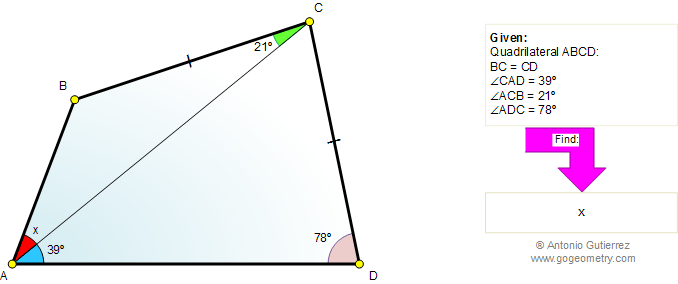Problem 556: Quadrilateral, Diagonal, Angles, Auxiliary Lines Level: High School, College, Math Education The figure shows a quadrilateral ABCD with diagonal AC and BC = CD. If angle CAD = 39 degrees, angle ACB = 21 degrees, and angle ADC = 78 degrees, find angle BAC.Geometry problem solving Geometry problem solving is one of the most challenging skills for students to learn. When a problem requires auxiliary construction, the difficulty of the problem increases drastically, perhaps because deciding which construction to make is an ill-structured problem. By “construction,” we mean adding geometric figures (points, lines, planes) to a problem figure that wasn’t mentioned as "given." Recent Additions